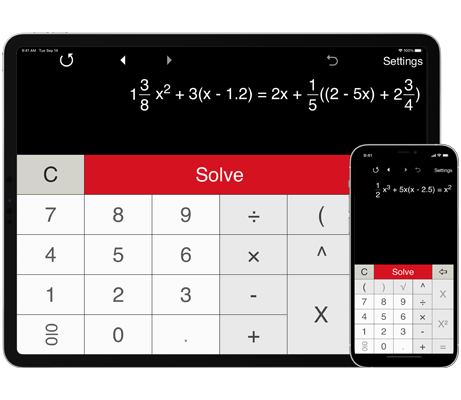# Equation Solver - quick help with equation problems

We believe math apps should be lightweight, powerful and universal. That’s why we created all-new, intelligent and multi-functional equation calculator 4in1 that helps you find solutions to various equation problems.
Over the last few weeks, we have been working to prepare this math solution to solve linear and quadratic equations step-by-step as well as cubic and quartic equations.

## Math problems solver for anyone who struggles with equations

With Equation Solver 4-in-1, equation calculation is easier than ever. With different modes to input equations, detailed step-by-step explanations for first degree and second degree equations and advanced history option the app becomes a great solution for learning and explaining equation problems you don't understand. Learn how Equation Solver is delivering a new way to solve equations

### More than an equation calculator

Supports 1st degree, 2nd degree, 3rd and 4th degree equations.
Solve a wide range of equations, including equations with brackets, parentheses and fractions.
Easily view and edit recently solved equations.
Print and share equations you solved.

#### Learn and practice

Whether you have to learn something new such as a quadratic equation or just need to refresh your memory on linear equations, this is the best app to do it.

Looking for more math homework help? Try our advanced calculator for fractions - Fractions Pro.

#### You can help us make the app better

Tell us what you think about the Equation Solver tool, what can be improved and what new features you'd like to see in new versions.